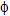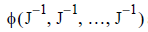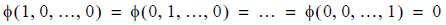# 5.4.5 - Decision Tree SQL Generation - Teradata Warehouse Miner

## Teradata Warehouse Miner User Guide - Volume 3Analytic Functions

Product
Release Number
5.4.5
Published
February 2018
Language
English (United States)
Last Update
2018-05-04
dita:mapPath
yuy1504291362546.ditamap
dita:ditavalPath
ft:empty

A key part to the design of the Teradata Warehouse Miner Decision Trees is SQL generation. In order to avoid having to extract all of the data from the RDBMS, the product generates SQL statements to return sufficient statistics. Before the model building begins, SQL is generated to give a better understanding of the attributes and the predicted variable. For each attribute, the algorithm must determine its cardinality and get all possible values of the predicted variable and the counts associated with it from all of the observations. This information helps to initialize some structures in memory for later use in the building process.

The driving SQL behind the entire building process is a SQL statement that makes it possible to build a contingency table from the data. A contingency table is an m x n matrix that has m rows corresponding to the distinct values of an attribute by n columns that correspond to the predicted variable’s distinct values. The Teradata Warehouse Miner Decision Tree algorithms can quickly generate the contingency table on massive amounts of data rows and columns.

This contingency table query allows the program to gather the sufficient statistics needed for the algorithms to do their calculations. Since this consists of the counts of the N distinct values of the dependent variable, a WHERE clause is simply added to this SQL when building a contingency table on a subset of the data instead of the data in the whole table. The WHERE clause expression in the statement helps define the subset of data which is the path down the tree that defines which node is a candidate to be split.

Each type of decision tree uses a different method to compute which attribute is the best choice to split a given subset of data upon. Each type of decision tree is considered in turn in what follows. In the course of describing each algorithm, the following notation is used:
1. t denotes a node
2. j denotes the learning classes
3. J denotes the number of classes
4. s denotes a split
5. N(t) denotes the number of cases within a node t
6. p(j|t) is the proportion of class j learning samples in node t
7. An impurity functionis a symmetric function with maximum value8. t1 denotes a subnode i of t
9. i(t) denotes node impurity measure
10. t1 and tR are the left and right split nodes of t